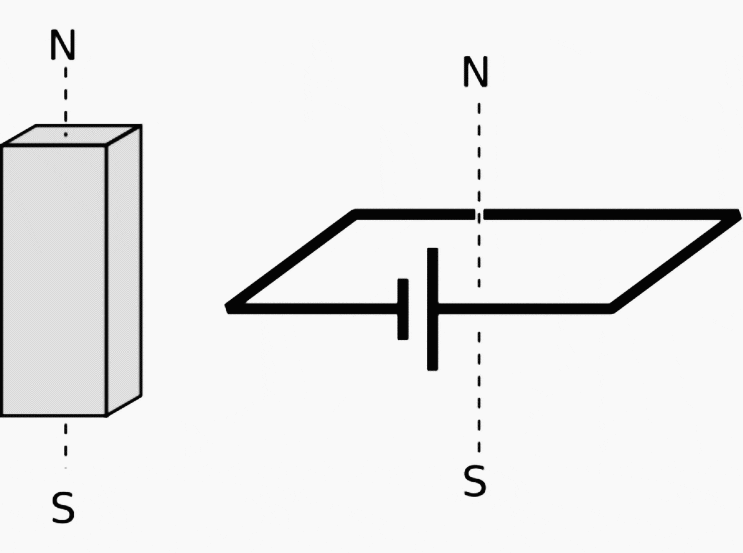Get access to premium HV/MV/LV technical articles, technical guides & papers. Apply a 20% discount code: 99AF8 for a 1-year plan!

# Electricity and magnetism textbook with exercises – Physics matters!

Home / Download Center / Electrical Engineering Books and Technical Guides / Electrical engineering guides / Electricity and magnetism textbook with exercises – Physics matters!

## Definition of the magnetic field

Since magnetic monopoles don’t seem to exist, it would not make much sense to define a magnetic field in terms of the force on a test monopole. Instead, we follow the philosophy of the alternative definition of the electric field, and define the field in terms of the torque on a magnetic test dipole.

This is exactly what a magnetic compass does: the needle is a little iron magnet which acts like a magnetic dipole and shows us the direction of the earth’s magnetic field.d / A – from a square loop of wire short- ing across a battery. It acts very much like a bar magnet, but its strength is more easily quantified.

To define the strength of a magnetic field, however, we need some way of defining the strength of a test dipole, i.e., we need a definition of the magnetic dipole moment. We could use an iron permanent magnet constructed according to certain specifications, but such an object is really an extremely complex system consisting of many iron atoms, only some of which are aligned.

A more fundamental standard dipole is a square current loop. This could be little resistive circuit consisting of a square of wire shorting across a battery.

We will find that such a loop, when placed in a magnetic field, experiences a torque that tends to align plane so that its face points in a certain direction. (Since the loop is symmetric, it doesn’t care if we rotate it like a wheel without changing the plane in which it lies).

It is this preferred facing direction that we will end up defining as the direction of the magnetic field.

Experiments show if the loop is out of alignment with the field, the torque on it is proportional to the amount of current, and also to the interior area of the loop. The proportionality to current makes sense, since magnetic forces are interactions between moving charges, and current is a measure of the motion of charge.

The proportionality to the loop’s area is also not hard to understand, because increasing the length of the sides of the square increases both the amount of charge contained in this circular “river” and the amount of leverage supplied for making torque.

Two separate physical reasons for a proportionality to length result in an overall proportionality to length squared, which is the same as the area of the loop. For these reasons, we define the magnetic dipole moment of a square current loop as:

Dm = IA
[definition of the magnetic dipole moment of a square current loop]

We now define the magnetic field in a manner entirely analogous to the second definition of the electric field:

The magnetic field vector, B, at any location in space is defined by observing the torque exerted on a magnetic test dipole Dmt consisting of a square current loop. The field’s magnitude is |B| = τ/Dmt sinθ, where θ is the angle by which the loop is misaligned.

The direction of the field is perpendicular to the loop; of the two perpendiculars, we choose the one such that if we look along it, the loop’s current is counterclockwise.

We find from this definition that the magnetic field has units of N · m/A · m2 = N/A · m. This unwieldy combination of units is abbreviated as the tesla, 1 T = 1 N/A·m. Refrain from memorizing the part about the counterclockwise direction at the end.

The nonexistence of magnetic monopoles means that unlike an electric field, h/1, a magnetic one, h/2, can never have sources or sinks. The magnetic field vectors lead in paths that loop back on themselves, without ever converging or diverging at a point.